# 什么是几何非线性？

2015年 9月 14日

### 考虑几何非线性的影响

• 空间 框架和 材料 框架之间进行了区分。由于存在位移矢量（\mathbf u），某一点的空间坐标（\mathbf x）与材料坐标（\mathbf X）不同，即 \mathbf x = \mathbf X + \mathbf u。因此，在表达式中使用大写或小写坐标名称将非常重要。
• 应变由格林-拉格朗日应变张量代替工程应变来表示。
• 应力由第二类 Piola-Kirchoff 应力张量表示。
• 压力载荷考虑了变形。边界的法线将更新，并且考虑拉伸引起的面积变化。

X– 方向的格林-拉格朗日法向应变可写为

\epsilon_X = \frac{\partial u}{\partial X} + \frac{1}{2}(\frac{\partial u}{\partial X})^2 + \frac{1}{2}(\frac{\partial v}{\partial X})^2 + \frac{1}{2}(\frac{\partial w}{\partial X})^2

\epsilon_X = \frac{\partial u}{\partial X}

\epsilon_{XY} = \frac{1}{2}(\frac{\partial u}{\partial Y} + \frac{\partial v}{\partial X} + \frac{\partial u}{\partial X} \frac{\partial u}{\partial Y} + \frac{\partial v}{\partial X} \frac{\partial v}{\partial Y} + \frac{\partial w}{\partial X} \frac{\partial w}{\partial Y} )

\epsilon_{XY} = \frac{1}{2}(\frac{\partial u}{\partial Y} + \frac{\partial v}{\partial X})

### 明显（或不太明显）的旋转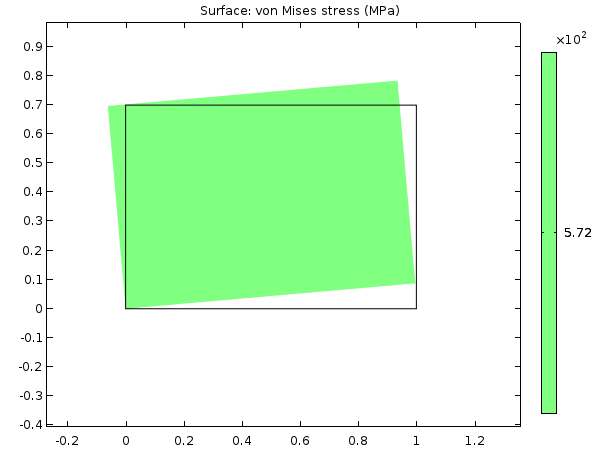\begin{matrix}
x = X \cos(\phi)-Y \sin(\phi) \\
y = X \sin(\phi) + Y \cos(\phi)
\end{matrix}

\begin{matrix}
u = x-X = X (\cos(\phi)-1)-Y \sin(\phi) \\
v = y-Y = X \sin(\phi) + Y (\cos(\phi)-1)
\end{matrix}

\begin{array}{l}
\epsilon_X = \frac{\partial u}{\partial X} = \cos(\phi)-1 \\
\epsilon_Y = \frac{\partial v}{\partial Y} = \cos(\phi)-1 \\
\epsilon_{XY} = \frac{1}{2}(\frac{\partial u}{\partial Y}+\frac{\partial v}{\partial X}) = -\sin(\phi)+ \sin(\phi) = 0
\end{array}

\epsilon_X = \frac{\partial u}{\partial X} + \frac{1}{2}(\frac{\partial u}{\partial X})^2 + \frac{1}{2}(\frac{\partial v}{\partial X})^2 = \cos(\phi)-1 + \frac{1}{2}(\cos(\phi)-1)^2+ \frac{1}{2}(\sin(\phi))^2 = 0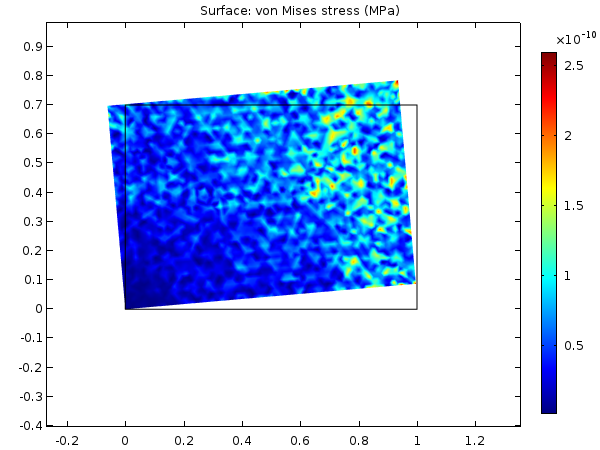### 薄结构拉伸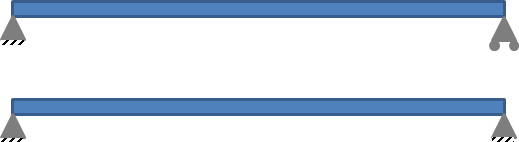• 当末端自由轴向移动时，梁的垂直位移几乎与几何线性情况相同。
• 当限制轴向位移时，垂直位移将小于线性情况，并且对载荷具有很强的非线性依赖性。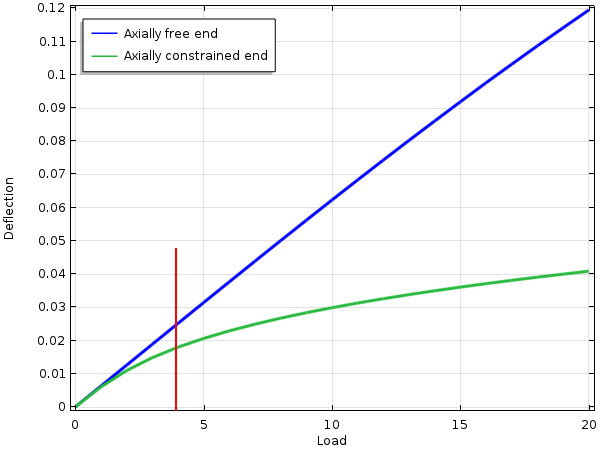### 应力刚化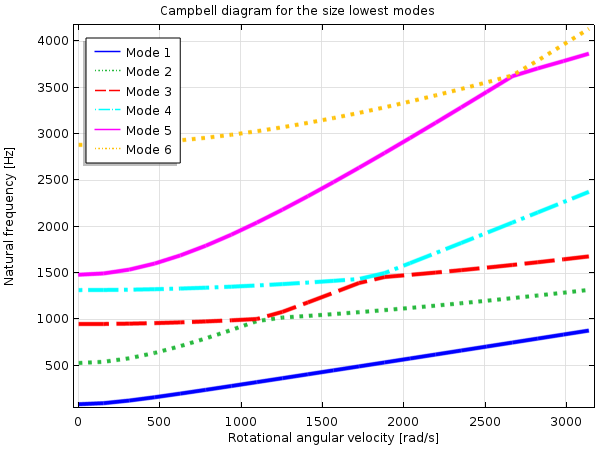1. 预应力分析，特征频率
2. 预应力分析，频域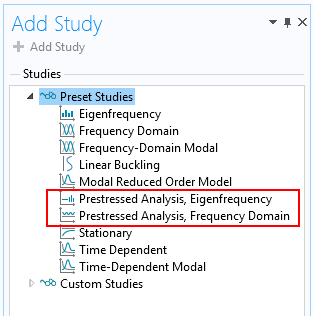### 屈曲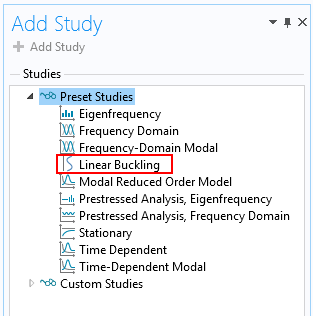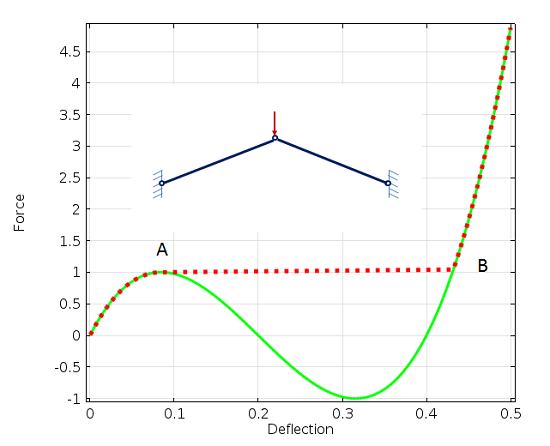### 在 COMSOL Multiphysics 中启用几何非线性

#### 启用几何非线性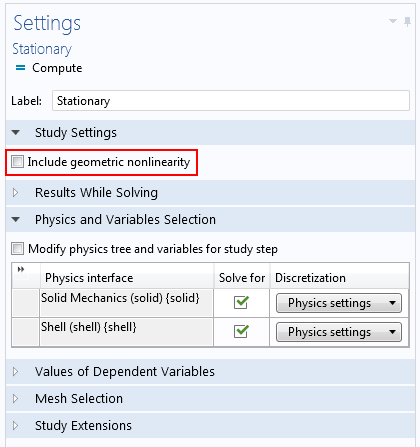• 超弹性材料
• 大应变塑性
• 接触

#### 求解几何非线性问题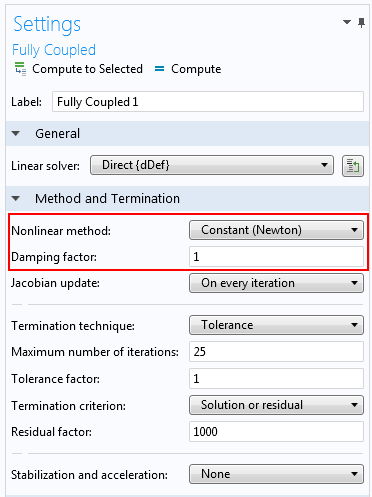COMSOL 案例库中的夹紧的半球壳教程模型中显示了如何设置求解器来解决严重非线性问题的示例。

### 结束语

• 即使非线性影响很小，调用非线性求解器也会带来更长的求解时间。对于小型模型而言，这不是问题，但是当使用数百万个自由度进行求解时，将求解时间减少两倍确实很重要。
• 有时我们希望能够与解析解进行比较，而此类解通常基于线性理论。
• 我们可能需要遵循假定使用线性方法的标准或分析过程。
• 在几何非线性问题中，必须使用实际载荷。如果我们只想对结构响应进行概念研究，那么如果估算的载荷太大，则解可能不会收敛。